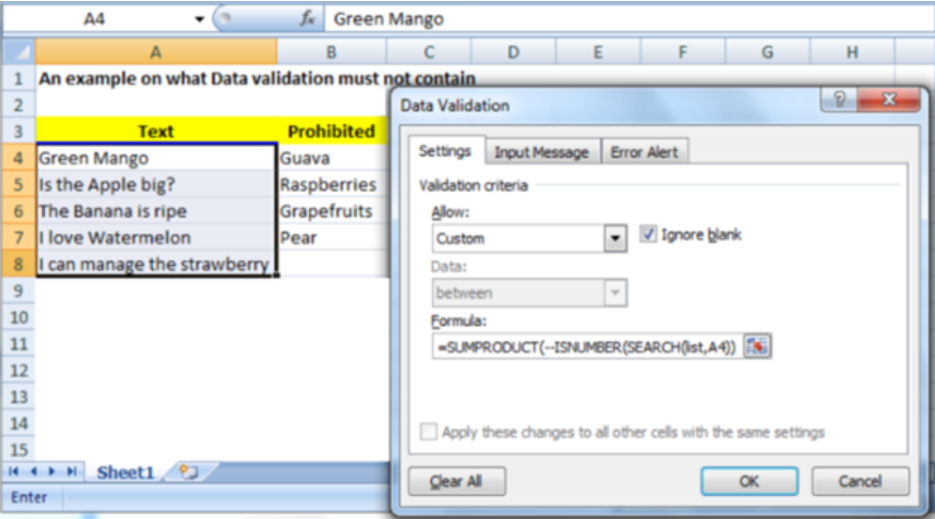Get instant live expert help with Excel or Google Sheets“My Excelchat expert helped me in less than 20 minutes, saving me what would have been 5 hours of work!”

#### Post your problem and you’ll get expert help in seconds.

Your message must be at least 40 characters
Our professional experts are available now. Your privacy is guaranteed.

# Data validation must not containRead time: 15 minutes

In excel, we can easily disallow an input which contains one of many things. To do this, we can use a validation rule which utilizes the SEARCH function. In this article, we shall look at how one can carry out data validation must not contain something else in excel.Figure 1: Data validation in Excel

# General syntax of the formula

`=SUMPRODUCT (--ISNUMBER (SEARCH (list, column to validate)))=0`

## Understanding the formula

The formula above plays a fundamental role when we want to disallow inputs that contain one or many things.

## How the formula works

• Data validation formula utilizes the SEARCH function when testing an input for each value that are in the list.
• The function uses the logic “contains”
• In the event that a value is found in the list, the SEARCH function will return its position as a number.
• When no value is found, the SEARCH function will return an error.
• The numbers will then be converted to TRUE while the errors to FALSE by the ISNUMBER function.
• These TRUE and FALSE strings are then converted to 1s and 0s by the double negative operator.
• These items are then summed up by the SUMPRODUCT function and then the result is tested against zero.
• Validation will occur if all items are zero and the SUMPRODUCT returns a zero. But if it returns any other number, then a FALSE is returned, meaning validation has failed.

Cell references when doing data validation applies to the upper left cell in the range that you have selected as of the moment the data validation rule is being entered.

## Instant Connection to an Expert through our Excelchat Service

Most of the time, the problem you will need to solve will be more complex than a simple application of a formula or function. If you want to save hours of research and frustration, try our live Excelchat service! Our Excel Experts are available 24/7 to answer any Excel question you may have. We guarantee a connection within 30 seconds and a customized solution within 20 minutes.

### Did this post not answer your question? Get a solution from connecting with the expert.Another blog reader asked this question today on Excelchat:
Solution examplesUse the Vlookup Function to complete the "employee" column of table 2. Use "job Id" from table 2 as your lookup_value(s) and table 1 as your reference.
Solved by C. H. in 16 minsIf a cell in another sheet is populated I need a vlookup done. If the cell is not populated I need the cell to return blank.
Solved by T. D. in 60 minsI am trying to make a chart that turns a week range red if nothing is entered in the range. If something is entered then I would like it to turn green. Please Help
Solved by E. U. in 43 minsI need a check box to show/hide an answer of an if function
Solved by Z. U. in 23 minsI want to display a range of cells if they meet a specific criteria. I want to display a name IF it does not equal one of three options AND there are any numbers contained in a range of cells
Solved by G. F. in 60 mins## Subscribe to Excelchat.coAnother blog reader asked this question today on Excelchat: